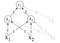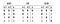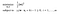# Support Vector Machines — Lecture series — Karush-Kahn-Tucker conditions part 4

In the previous post, we looked at what primal and dual problems were. In this post, we will be looking at a theorem which expresses the inferences that we can derive from the relationship between the dual and the primal linear programming problems. This theorem is called the ‘duality’ theorem.

Learning objective:

The main objective of this post is to gain an understanding of the duality theorem.

Main question:

Can we draw any conjectures from the relationship between primal problems and their corresponding dual problems?

The answer to this question is yes and the duality theorem aids us in doing…

# Natural Language Processing — Neural Networks and Neural Language Models Lecture series — Feed-Forward Neural Networks

In this post, we will be learning about the concept of feed-forward neural networks and the mathematical representation of this concept.

Feed-Forward Neural Network:

A feed-forward neural network is simply a multi-layer network of neural units in which the outputs from the units in each layer are passed to the units in the next higher layer. These networks do not have any cycles within them. That is, the outputs within the network do not flow in a cyclical manner.

To gain a refreshed understanding of what neural units are and how they work, you can read about them here.

Graphical…

# Support Vector Machines — Lecture series — Karush-Kahn-Tucker conditions part 3

In the previous post, I explained the various what the various KKT conditions were about with the exception of the ‘complementary slackness’ condition. In the subsequent series of posts, I intend to break down this concept to enable you to have maximum understanding. To begin this quest, I will be first be talking about the concept of ‘Primal’ and ‘Dual’ linear programming problems.

Learning objective:

To have an understanding of the complementary slackness condition, I will first be explaining the concept of ‘Primal’ and ‘Dual’ problems.

Main question:

What are the ‘primal’ and ‘dual’ natures of linear programming problems?

Consider…

# Natural Language Processing — Neural Networks and Neural Language Models Lecture series — The XOR problem part 2

In the previous post, we spoke about the XOR problem and we also mentioned how neural networks could be used to solve the XOR problem. In this post, we will be talking about how we can formulate a neural network structure to solve the XOR problem.

The neural network structure that we will be discussing was formulated by Goodfellow and it is demonstrated in Fig. 1 below:Fig. 1

The architecture of the neural network in Fig. 1 is made up of an input layer, consisting of x1 and x2, a middle layer, consisting of h1 and h2, and an output layer…

# Support Vector Machines — Lecture series — Karush-Kahn-Tucker conditions part2

In the previous post, we looked at the various conditions that make up the Karush-Kahn-Tucker (KKT) conditions. In this post, we would explore each of those conditions and explain what they mean.

Learning objective:

Understand the main ideas behind the various KKT conditions.

Main questions:

What do the following KKT conditions mean:

1. The stationary condition
2. The prime feasibility condition
3. The dual feasibility condition
4. The Complementary slackness condition

The stationary condition:

The stationary condition just states that the selected point must be a stationary point. A stationary point refers to the point at which the function stops increasing or decreasing. When…

# Support Vector Machines — Lecture series — Karush-Kuhn-Tucker conditions part 1

In the previous post, we spoke about the Wolfe dual problem which simply expressed another way in which we could express the SVM optimisation problem. However in this post, we will be looking at the conditions that need to be met before we can declare that the solution obtained from solving this optimisation problem is optimal in nature. These conditions are referred to as Karush-Kuhn-Tucker (KKT) conditions. They are referred to as “Karush-Kuhn-Tucker" conditions because they were first published in 1951 by Harold W. Kuhn and Albert W. …

# Support Vector Machines — Lecture series — The Wolfe dual problem

In the previous post, we spoke about how hard it was to solve an SVM optimisation problem in the initial way in which it was expressed. We also spoke about how to re-write the optimisation problem into a way in which it would be much easier to solve. The alternative that we suggested involved looking at the SVM optimisation problem through the lens of a ‘min max’ problem instead of through the lens of just a ‘min’ problem. …

# Natural Language Processing — Neural Networks and Neural Language Models Lecture series — The XOR problem

In this post, we will be learning about the XOR problem and how it can be solved.

Consider the following image in Fig. 1:Fig. 1

The XOR problem is that, we can formulate perceptrons that could take in as inputs the values of x1 and x2 for the operations of AND and OR and generate their corresponding y outputs but it would be impossible to formulate a perceptron that could take in as inputs the values of x1 and x2 and then generate their corresponding y outputs for the operation of XOR.

Note: A perceptron is a neural unit that has…

# Support Vector Machines — Lecture series — The SVM Lagrangian problem part 2

In the previous post, I spoke about how to apply the concept of Lagrange multipliers to solve an SVM optimisation problem. In that post, I spoke about how hard it was to solve the derived Lagrange function for an example SVM optimisation problem. In this post, we will be talking about why it is hard to solve such a problem and we will be talking about how to go about solving such a problem.

Learning objective:

Understand how hard it is to solve a Lagrange function for an SVM optimisation problem and how to go about solving such a problem.

# Support Vector Machines — Lecture series — The SVM Lagrangian problem

In the previous post, we talked about how to apply the concept of Lagrange multipliers to solve optimisation problems. In this post, we will be talking about how to apply the concept of Lagrange multipliers to solve an SVM optimisation problem.

Learning objective:

Understand how to apply Lagrange multipliers to solve SVM optimisation problems.

Main question:

How would you apply the concept of Lagrange multipliers to solve the SVM optimisation depicted in Fig. 1 below:Fig. 1

Well, the first step would be to introduce a Lagrange function. Like what is depicted in Fig. 2 below: